# A FPGA Based PI Adaptive Sliding Mode Controller for PEM Fuel Cell with Boost Converter

A FPGA Based PI Adaptive Sliding Mode Controller for PEM Fuel Cell with Boost Converter
School of Mechanical Engineering, Nantong Institute of Technology, Nantong 226002, China

School of Mechanical Engineering, Nantong Institute of Technology, Nantong 226002, China

School of Mechanical Engineering, Nantong Institute of Technology, Nantong 226002, China

Corresponding Author Email:
gaojie.ntit@gmail.com
Page:
179-187
|
DOI:
https://doi.org/10.14447/jnmes.v25i3.a04
5 May 2021
|
Accepted:
12 July 2022
|
Published:
31 August 2022
| Citation

OPEN ACCESS

Abstract:

Proton exchange membrane (PEM) is one of the most popular fuel cells for renewable energy production, and this paper presents a DC/DC boost converter structure to improve energy efficiency. To achieve high output power as well as constant output voltage, a combined method consisting of proportional-integral controller and adaptive sliding mode technique is designed. Also, due to the use of adaptive technique, the proposed technique has the ability to cover the effects of uncertainty with an unknown high limit and in addition to improving the quality of output power and voltage of PEM, it eliminates permanent tracking error and guarantees closed loop system stability. To show the operational implementation capability and flexibility of the method, FPGA has been used, which in addition to showing real-time performance, provides the ability to execute the controller at high speeds. Operationality and the possibility of practical implementation of the planned scheme on the existing systems provide the possibility of increasing the efficiency of energy extraction without further investment. The stability of the closed-loop system is achieved using Lyapunov technique and the results of simulation and comparison indicate the optimal performance of the system under the planned scheme and high efficiency in comparison with existing approaches.

Keywords:

FPGA; fuel cell; boost converter; PI adaptive sliding mode control; uncertainty

1. Introduction

Energy is the most important need and subject of research in today's world [1-4], and many researches are being carried out in the field of different techniques of its production from different sources [5-6], proper storage [7-8], its use in various applications [9-10], and its management and control [11 12]. Efforts to prevent devastating changes in climate and the environment have led to modifications in the energy sector, and achieving global goals in this area is possible with the improvement of low-carbon energy expertise, especially with the use of renewable energy. The energy sector is the main producer of greenhouse gases and the main cause of global warming, and change in the energy production system is seen as the key to achieving a cleaner and safer future. Renewable energies, in addition to being clean, are abundant and reliable, and if properly developed, can play an important role as a sustainable energy source in meeting the needs and reducing climate change [13-16].

One of the most significant expertise being developed for the generation of renewable energy is fuel cells. These devices operate on the principles of electrochemistry, and one of the most prevalent kinds is the proton exchange membrane fuel cell (PEMFC). This tools provides a safe, clean and efficient energy source with a long life and costs much less than other fuel cell technologies . In addition, PEM has a wide variety of applications in transportation, vehicles and distributed power generation because of its high power density, low temperature in service and high conversion efficiency.

The DC/DC converters are often used to convert the output power of the fuel cell to the appropriate usable power. There are various techniques in using converters, the most popular of which are buck converters (voltage reducers), boost converters (voltage boosters) and other types of converters such as buckboost and Cuk . In these converters, DC output voltage is controlled through pulse width modulation (PWM) technique.

To achieve greater efficiency and transfer as much power as possible from the fuel cell stack to the load, different control techniques have been used to ensure that the system operates at the maximum power point. Factors such as nonlinearity of the fuel cell system, uncertainty in the model, and disturbances seriously affect the performance of classical linear control approaches for DC/DC converters. For this reason, recently advanced techniques such as feedback linearization , fuzzy logic controller (FLC) [20, 21] and sliding mode controller (SMC) [22-25] have been widely considered in articles. Artificial neural network (ANN) and particle swarm optimization (PSO) techniques have also been used to increase the efficiency of control approaches. Sliding mode controller has been used in various fields due to several advantages such as the ability to stabilize the system against changes in the converter parameters and load disturbances [26-28], ease of operation and excellent performance [29-30]. However, applying a sliding mode technique in a DC-DC converter causes some major difficulties including: 1. Change the switching frequency and create low-order harmonic components; 2. Steady state error; 3. The overall system instability resulting from a right zero in the voltage transfer function of DC-DC boost converter; 4. Finally, in addition to not considering the effects of parasitic and destructive elements on the converter model, the possibility of practical implementation of the proposed method has been completely neglected.

To overcome the stated issues and getting the maximum output power from the PEM fuel cell, this paper schemes a robust indirect technique for controlling the PEM output voltage with the possibility of its practical implementation. Using proportional-integral (PI) control method, sliding mode and adaptive techniques, a hybrid scheme has been planned to control the output voltage of PEM fuel cell, which in addition to stabilizing the system in the operating range, is able to cover parametric uncertainty effects. The proposed innovative approach is implemented using Field Programmable Gate Arrays (FPGAs) which is used as a suitable platform for embedded control systems and provides a suitable hardware substrate with advantages such as high execution speed, design Offers, high flexibility and reliability. It also allows the designer to test and validate the control algorithm and optimize its performance in less time and at less cost without real and practical implementation. Given the advantages of FPGA in various areas of performance, reliability, long-term maintenance, cost and time to market, this paper presents a new hybrid control method implemented through FPGA to improve the energy efficiency of PEM fuel cells.

So, the paper has been compiled as follows. The second part presents the PEM fuel cell model equipped the DC-DC converter. The third section describes the planned FPGA based controller configuration. In the fourth section, the results of simulations and comparisons have given by using of Toolbox FPGA. Finally, the fifth part is devoted to conclusions and future proposals.

2. Model of Fuel Cell System Model with DC-DC Boost Converter

This part describes the fuel cell system model with DC-DC boost converter. The arrangement of static and dynamic representations of PEM fuel cell in company with the general model of fuel cell with converter are given in three sections.

2.1 The static model of PEM fuel cell

Converting chemical energy into electrical energy is the main task and function of PEM fuel cell, so its overall plan is shown in Figure 1.

## p1.png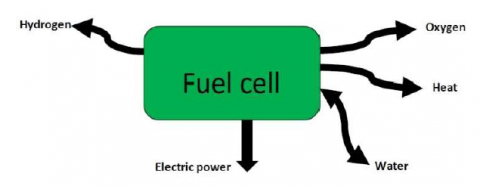Figure 1. Overall plan of PEM fuel cell

In the energy conversion process inside the fuel cell, ordinary water and heat are produced. The different parts of the fuel cell, containing anode, cathode and membrane, are presented in Figure 2. The oxidation reaction inside the fuel cell is as follows:

$2 \mathrm{H}_2 \Longrightarrow 4 \mathrm{H}^{+}+4 e^{-}$          (1)

The free $\mathrm{H}^{\wedge}+$ ions pass through the membrane, while the $e^{\wedge}-$ ions cannot. The subsequent reduction reaction takes place inside the cathode as well.

$\mathrm{O}_2+4 \mathrm{H}^{+}+4 e^{-} \Rightarrow 2 \mathrm{H}_2 \mathrm{O}$          (2)

In reaction (2), oxygen entering the cathode reacts with $\mathrm{H}^{\wedge}+$ moving from side to side the membrane and $e^{\wedge}-$ is also transferred to the surface of the cathode with an external load. The overall reaction is as follows:

$2 \mathrm{H}_2+\mathrm{O}_2 \Rightarrow 2 \mathrm{H}_2 \mathrm{O}+$ electrical energy          (3)

Therefore, the output voltage of single fuel cell $\left(\mathrm{V}_{-} \mathrm{Fc}\right)$ and stack fuel cell (V_stack) will follow the relations (4) and (5).

$V_{F c}=E_{\text {Cell }}-\eta_{a c t}-\eta_{o h m}-\eta_{\text {con }}$           (4)

$V_{\text {stack }}=N_{\text {cell }} \cdot V_{F c}=N_{\text {cell }} \cdot\left(E_{c e l l}-\eta_{a c t}-\eta_{o h m}-\eta_{\text {con }}\right)$          (5)

In the mentioned equations, E_Cell indicates the PEMFC electrochemical thermodynamics potential and it reports the best yield voltage, $\eta_{-}$act specifies activation polarization loss and identifies the voltage drop due to the activation of the anode and the cathode, $\eta$_ohm indicates ohmic polarization loss resulting from membrane resistance to transfer protons and from electrical resistance of the electrodes to transfer electrons, $\eta_{-} \operatorname{con}$ provides the loss of concentration polarization caused by the decrease in the concentration of the reactants, oxygen and hydrogen, which varies during the reaction and N_cell specifies the number of fuel cell in stack.

## p2.png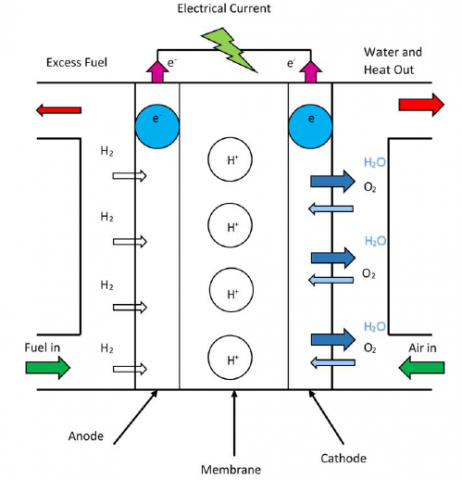Figure 2. Different sections of PEMFC and their operation diagram

The dynamic model of PEMFC is given in this part. For the reason that the membrane is placed in the mid of two charged layers in the PEMFC, it works as a capacitor in point of fact. Since $\mathrm{R}_{\mathrm{ohm}}$, $\mathrm{R}_{\mathrm{act}}$, $\mathrm{R}_{\mathrm{con}}$ are ohmic, activation and the concentration resistances respectively, the PEMFC equivalent circuit is given as in Figure 3.

By considering the Kirchhoffs Voltage Law (KVL) and Kirchhoffs Current Law (KCL) for the equivalent circuit, it is achieved

$V_{\text {cell }}=E_{\text {cell }}-V_d-I R_{\text {ohm }}$       (6)

$I=C \frac{d V_d}{d t}+\frac{V_d}{R_{c o n}+R_{a c t}}$       (7)

## p3.png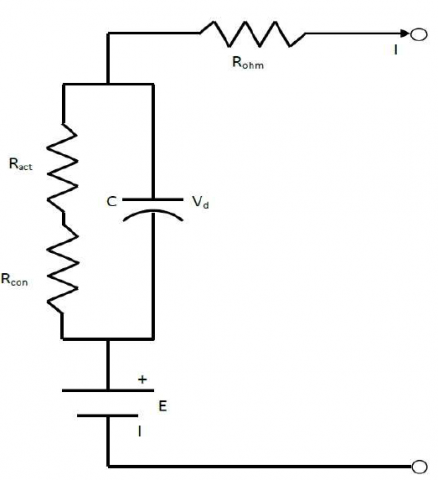Figure 3. The equivalent circuit of PEMFC

Equation (7) can be rephrased in the following way:

$\frac{d V_d}{d t}=\frac{1}{C} I-\frac{1}{\tau} V_d$        (8)

Where $V_d$ states the voltage through the equivalent capacitor, $C$ is the corresponding capacitance, and $\tau$ specifies the fuel cell time constant as following:

$\tau=C\left(R_{a c t}+R_{c o n}\right)=C\left(\frac{\eta_{a c t}+\eta_{c o n}}{I}\right)$         (9)

By inserting equations (9) and (8) into equation (6) and taking the Laplace transform, it is obtained

$V_{\text {cell }}=E_{\text {cell }}-\left(\frac{R_{\text {act }}+R_{\text {con }}}{\left(R_{\text {act }}+R_{\text {con }}\right) C . s+1}+R_{\text {ohm }}\right) I$          (10)

To describe the behavior of PEM more accurately, the pressure of hydrogen and oxygen gases as well as their flow should be included in the dynamic model. According to references [31-32], partial pressure equations for oxygen and hydrogen are as follows:

\begin{aligned} P_{H_2}=\frac{1 / K_{H_2}}{\left(1+\tau_{H_2}\right)} &\left(q_{H_2}-2 I \cdot K_r\right) 2 H_2 \\ & \Rightarrow 4 H^{+}+4 e^{-} \end{aligned}          (11)

$P_{O_2}=\frac{1 / K_{O_2}}{\left(1+\tau_{O_2}\right)}\left(q_{O_2}-I \cdot K_r\right)$          (12)

Where:

\left\{\begin{aligned} \tau_{H_2} &=\frac{V_{a n}}{R \cdot T \cdot K_{H_2}} \\ \tau_{O_2} &=\frac{V_{a n}}{R \cdot T \cdot K_{O_2}} \end{aligned}\right.            (13)

$K_{H_2}$ is the valve molar constant of hydrogen (kmol/s atm), $K_{O_2}$ means the valve molar constant of oxygen ( kmol/ s atm), $q_{H_2}$ specifies the flow rate of hydrogen, $q_{O_2}$  is the oxygen flow rate, $\tau_{H_2}$ indicates the response time of hydrogen (s), $\tau_{O_2}$ is the response time of oxygen (s), $K_r$ means a modeling parameter constant $(\mathrm{kmol} /(s A))$, and $V_{a n}$ is the anode volume. By means of the mentioned equations, the PEMFC system can be arranged as Figure 4.

## p4.png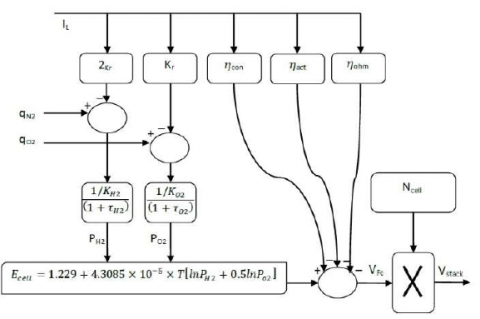Figure 4. Dynamic model of PEMFC system

2.2 The state space model of DC/DC boost converters

The duty of the PEMFC DC/DC booster converter is to increase the voltage level and its plan is given in Figure 5.

## p5.png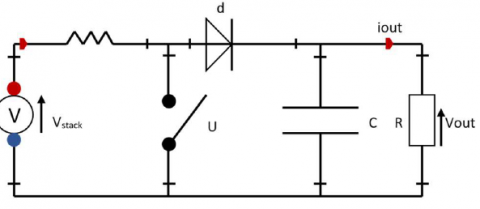Figure 5. The plan of PEMFC DC/DC booster converter

This plan includes a PEM as a voltage source, a transistor switch, a diode switch, a filter capacitor, an inductance and an ohmic load. Through the $u$ switch, the output follows the subsequent relationship

$V_{o u t}=\left(\frac{1}{1-u}\right) \cdot V_{s t a c k}$          (14)

The working modes of this boost converter are in two ways, in the first mode, when $u$ is on and $d$ is off, the dynamic equations of the inductance current and output voltage are like so:

$\left\{\begin{array}{l}\frac{d i_L}{d t}=\frac{1}{L}\left(V_{\text {stack }}\right) \\ \frac{d V_{\text {out }}}{d t}=\frac{1}{C}\left(-i_{\text {out }}\right)\end{array}\right.$    (15)

In the second operating manner, when $u$ is off and $d$ is on, the dynamic equations of inductance current and output voltage are like this:

$\left\{\begin{array}{l}\frac{d i_L}{d t}=\frac{1}{L}\left(V_{\text {stack }}-V_{\text {out }}\right) \\ \frac{d V_{\text {out }}}{d t}=\frac{1}{C}\left(i_L-i_{\text {out }}\right)\end{array}\right.$     (16)

By defining inductance current and output voltage as state variables and also $V_{\text {stack }}=v$, the state space equations for the boost converter are:

$\left\{\begin{array}{c}\dot{x}=\left(\begin{array}{cc}0 & \frac{u-1}{L} \\ \frac{1-u}{C} & -\frac{1}{R C}\end{array}\right) x+\left[\begin{array}{l}\frac{1}{L} \\ 0\end{array}\right] v \\ y=\left[\begin{array}{ll}0 & 1] x\end{array}\right.\end{array}\right.$    (17)

Table 1. The parameters of PEMFC

 Symbol Symbol Define Value Unit $E_o$Reference potential (in volts) $1.229$ $V$$R$Gas constant $83.143$ $\mathrm{Jmol}{ }^{-1} K^{-1}$$F$Faraday constant $96485.309$ $\mathrm{Cmol}^{-1}$$T$membrane temperature used in the tests (in Kelvin) $298.15$ $K$$A$membrane active area 162 $\mathrm{cm}^2$$\psi$parametric coefficient, used to model the membrane resistance(minimum14, maximum 23) 23 $-$ $l$membrane thickness $175.10^{-6}$$\mathrm{cm}$$B$parametric coefficient, used in the calculation of the concentration losses $0.1$ $V$$R_c$membrane equivalent contact resistance $0.0003$ $\Omega$ $J_{\max }$ maximum current density $0.062$$\mathrm{A} \mathrm{cm}^{-2}$ $N_{\text {cell }}$ number of FCs used in the stack 10 $-$ $\xi_1$ $\xi_1 \ldots \xi_4$ are parametric coefficients, based on electrochemical, kinetics, and $0.9514$ $V$$\xi_2$ $-0.00312$ $V / K$ $\xi_3$ $-7.4 .10^{-5}$ $V / K$ $\xi_4$ $1.87 .10^{-4}$ $V / K$

3. Planned FPGA Based PI Adaptive Sliding Mode Controller

The planned control scheme is defined in this part. In the plan of the control technique, a number of problems have been reflected which have been neglected by previous studies: 1- Due to the non-minimum phase nature of the DC/DC boost converter, direct control approaches are not able to correctly control and extract the maximum power from the DC source, and of course this feature of the converter leads to controller error and instability of the overall system; 2- Like any other physical system, uncertainty in the system model is inevitable. These uncertainties occur in different parts of the system and sometimes their upper limit is not known; 3- The most important approach of this study is the possibility of implementing the planned technique through FPGA. This approach enables the practical realization of the planned technique and provides the possibility of its development to the existing systems.

To overcome the adverse effects stated above and of course the practical realization of the planned approach, this paper presents a new hybrid control skill based on PI, adaptive and sliding mode techniques with the possibility of implementation through FPGA. In order to achieve zero steady state error and extract maximum power from the fuel cell, the sliding mode scheme is combined with the PI technique. This approach guarantees zero error in the induced current and thus maximum power extraction. Also, by using the adaptive approach, the upper bound of uncertainties is estimated and the stability of the system is guaranteed in the conditions of uncertainty. Finally, the different parts of the controller are structured using the FPGA platform in the MATLAB environment. The general schematic of the controller is given in Figure 6.

Considering $\mathrm{V}_{\text {stack }}$, $\quad i_{\mathrm{ref}}$, $\quad i_L$ and $V_{\text {out }}$, the planned scheme performs the necessary control action on the boost converter and determines the desired $i_{\mathrm{L}}$ and $V_{\text {out }}$. Now the control design process is described.

## p6.png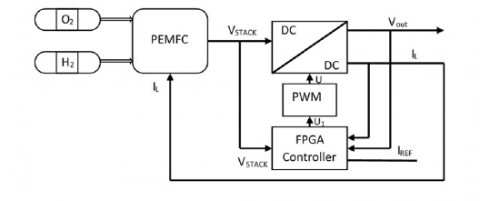Figure 6. The general schematic of controller for PEMFC power system

By applying the PI technique, which is realized by the FPGA platform in Figure 7, the dynamics of the output voltage is defined as follows:

## p77.png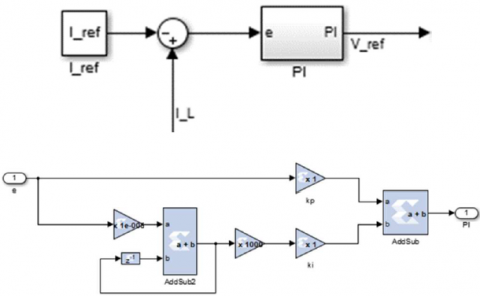Figure 7. Realization of PI technique through FPGA

$V_{r e f}=k_P e+k_I \int e d t$          (18)

Where $k_P$ and $k_I$ specify the PI controller gains and the state variables are described as

$e_1=i_L-i_{\text {ref }}$

$e_2=V_{\text {out }}-V_{\text {ref }}$          (19)

In relation to Equation (17), the dynamics of the error variables are

$\dot{e}_1=\frac{1}{L}\left(V-V_{o u t}\right)+\frac{1}{L} u$

$\dot{e}_2=\frac{1}{C} i_L-\frac{1}{R_C} V_{o u t}-\frac{k_P}{L}\left(V-V_{o u t}\right)$

$-k_I\left(i_L-i_{\text {ref }}\right)-\left(\frac{1}{C}+\frac{k_P}{L}\right) u$          (20)

Establishing the condition of stability by using the Lyapunov principle under the robust controller guarantees the condition of convergence of the system to the sliding surface. By selecting the sliding switching surface as

$S=k_P\left(i_L-i_{r e f}\right)+k_I \int\left(i_L-i_{r e f}\right) d t$          (21)

Fulfillment of the condition (22) for the sliding surface is required to achieve the control goal using the planned control scheme.

$\frac{d S}{d t}=0$          (22)

It is obtained by applying Equation (20) in Equation (22)

$\dot{S}=k_P\left(\frac{1}{L}\left(V-V_{\text {out }}\right)+\frac{1}{L} u\right)+k_I\left(i_L-i_{\text {ref }}\right)$

$=\psi(.)+u$          (23)

Where

$\psi(.)=k_I\left(i_L-i_{r e f}\right)+\frac{k_P}{L}\left(V-V_{\text {out }}\right)$

$+\frac{k_P-L}{L} u$          (24)

The Lyapunov technique is used to ensure stability and find out the suitable control signal to realize the control objectives. The candidate Lyapunov function is chosen as equation (25).

$V=\frac{1}{2} S^2+\frac{1}{2} \tilde{\psi}^2$          (25)

Where $\tilde{\psi}$ indicates the error between $\Psi$ and $\hat{\psi}$

$|\psi(.)|<\Psi$          (26)

Where $\Psi$ states the unknown upper limit of $\psi(.)$ and $\hat{\psi}$ specifies the upper limit estimation. Derived from the Lyapunov function (25) provides

$\dot{V}=S \dot{S}-\tilde{\psi} \dot{\hat{\psi}}$          (27)

By inserting equation (23) in equation (27), we have

$\dot{V}=S \psi(.)+S u-\tilde{\psi} \dot{\hat{\psi}}$          (28)

Now pick the control signal as follows:

$u=-k \operatorname{sign}(S)-\hat{\psi}$          (29)

It is now achieved by placing (29) in equation (28)

$\dot{V}=-k S \operatorname{sign}(S)+S(\psi(.)+\hat{\psi})-\tilde{\psi} \dot{\hat{\psi}}$          (30)

Consistent with Equation (40), Equation (44) can be modified as follows

$\dot{V} \leq-k|S|+S(\Psi-\widehat{\psi})-\tilde{\psi} \dot{\hat{\psi}}$           (31)

To find the adaptive rule, we modify Equation (31) as follows

$\dot{V} \leq-k|S|+|\tilde{\psi}|(S-\dot{\hat{\psi}})$           (32)

Consistent with (32), to eliminate adaptive error$\tilde{\psi}$, its coefficient should be zero. For this reason, the adaptive law is defined as follows

$\dot{\hat{\psi}}=\lambda S$          (33)

Where $\lambda>1$ specifies the parameter of adaptive law regulation. The realization of this part of the controller by FPGA platform is shown in Figure 8.

## p8.pngFigure 8. Realization of adaptive technique through FPGA platform

By placing equation (33) in (32), we have

$\dot{V} \leq-k|S|-(\lambda-1)|S||\tilde{\psi}|$          (34)

Equation (34) confirms that by control signal of Equation (29) and through adaptive rule (33), the closed loop system is stable and the realization of control goals is guaranteed.

The complete implementation of the planned FPGA based control method is given in Figure 9. A connection between Simulink MATLAB and the FPGA platform is established through the system generator and input and output gate blocks. In the next part, simulation in MATLAB and FPGA environments will be used to accurately evaluate the capabilities of the controller.

## p9.png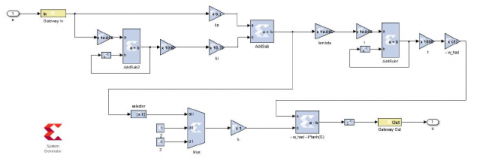Figure 9. The complete implementation of the planned FPGA based control method

4. Numerical Simulation Results

The aim of this part is to apply the planned control scheme on the system involving the PEMFC and DC/DC boost converter and MATLAB simulation environment and FPGA platform have been used to assess its performance. The subsequent hardware is used for this purpose. Intel Core i7- 10750H, 16GB DDR4 RAM, 512GB SSD, NVIDIA GeForce RTX 2060, 6GB GDDR6. Also, the results obtained from the application of the planned FPGA based scheme have been compared with the results from the implementation of supertwisting algorithm (STA) and SMC approaches from reference  in order to have a better analysis of the efficiency of the mentioned scheme. System parameters and operating conditions are all taken from .

As mentioned before, the aim of this study is the practical implementation of the controller through FPGA to extract the maximum possible power from PEMFC despite the nature of the non-minimum phase of the converter and also the presence of uncertainty in the load. For this purpose, extreme changes in the load according to Figure 10 are considered in the simulation. Also, according to , the current $i_l=9.74 \mathrm{~A}$ is considered as the reference current to guarantee the optimal performance of the controller, because the maximum power extraction occurs at this load current value. Now the obtained results are explained.

The output voltage under FPGA based, SMC and STA controllers is shown in Figure 11. Figures 12 and 13 also show the voltage of one PEM cell and a PEM stack under load changes and with different control approaches.

## 10.pngFigure 10. The extreme changes in the load used for simulation scenario

## 11.png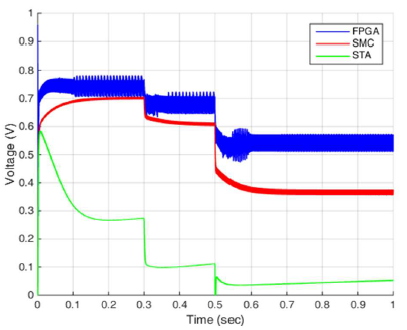Figure 11. The output voltage under FPGA based, SMC and STA approaches

## 12.png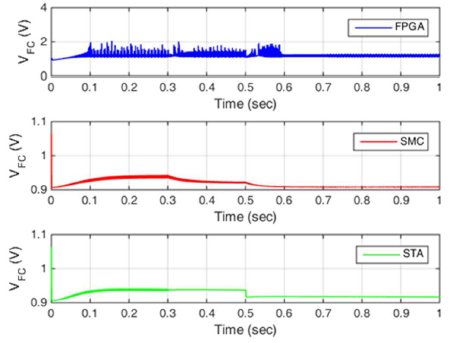Figure 12. One PEM fuel cell voltage under FPGA based, SMC and STA approaches

## 13.png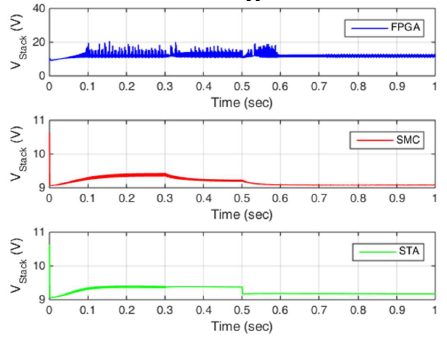Figure 13. The PEM fuel cell stack voltage under FPGA based, SMC and STA approaches

## 14.png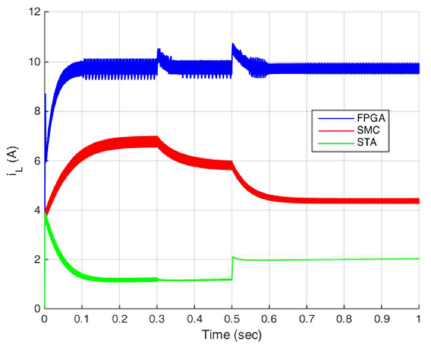Figure 14. Output current under FPGA based, SMC and STA approaches

The points that can be seen from Figure 14 are as follows: The phenomenon of chattering is inevitable in the results obtained from all three used control approaches, and according to the system structure and extreme changes in the load, the chattering phenomena, overshoot/undershoot and rapid changes  in  the  signals  are  observed.  Also,  only  planned technique  is  capable  of  tracking  the  reference  current $i_l$=9.74A ,  and  other  approaches  are  not  able  to  perform this tracking and ensure stability and getting optimal performance due to the nature of the converter and also the effects of uncertainty.

The output voltage changes of the boost converter by applying the planned FPGA based, SMC and STA approaches are shown in Figure 15. As it is clear from this figure at different moments, the output voltage of the converter in the FPGA-based method is about 1.7 times the SMC technique and 2.6 times the STA scheme. The changes in the output voltage of the boost converter according to the changes in the output load achieve the maximum power of the converter fuel cell structure are shown in Figure 16. The figure shows power extraction more than 2 times in FPGA based method compared to SMC technique and more than 8 times compared to STA scheme which of course can be justified by tracking the optimal load current by the planned technique and considering the output voltage diagram of the converter in Figure 15. As it is clear, despite the extreme load changes, the planned FPGA based hybrid scheme achieves the maximum power in the shortest possible time, and its robust performance is shown in Figure 16. The STA and SMC approaches are not able to provide the maximum power that can be extracted from the fuel cell system due to their inability to track the optimal current, and as a result, they cannot guarantee the robust and optimal performance of the system.

## 15.png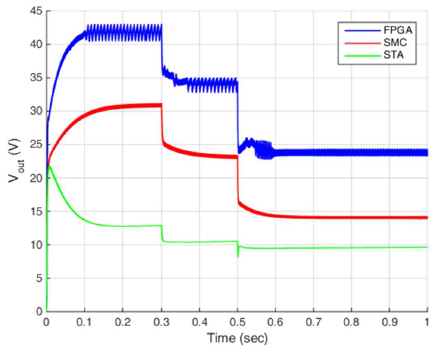Figure 15. The convertor output voltage under FPGA based, SMC and STA approaches

## 16.png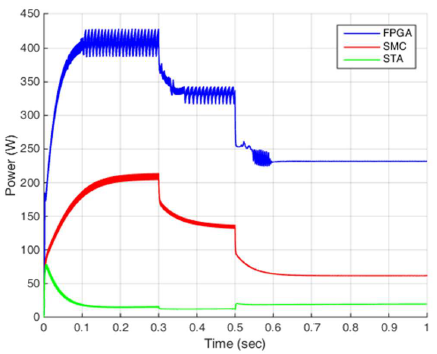Figure 16. The converter output power under FPGA based, SMC and STA approaches

5. Conclusion

Considering the importance of energy and the need to extract maximum power from renewable sources, a new hybrid control method for PEMFC system with DC/DC boost converter was presented in this study. Considering the non- minimum phase state of the converter as well as the uncertainty of the model, a new indirect hybrid control structure was planned using adaptive, sliding mode and PI techniques to extract the maximum power and its practical implementation was done by using FPGA user interface with MATLAB. Despite the non-minimum phase nature of the converter, the simulation results showed the ability to accurately track the optimal load current and, as a result, extract the maximum power from the fuel cell system, overcome the effects of uncertainty, and show the robust and optimal performance by applying the planned scheme. Considering the output power distortions under the planned hybrid technique, which is a weakness for the present study, the power extracted from the fuel cell system using the FPGA technique can be increased to more than twice the power under the previous procedures. Future studies will include the design of other robust control approaches to overcome the effects of chattering, considering the limitations of the control signal and cover the effects of disturbance and noise.

References

 Fan, S., Wang, X., Cao, S., Wang, Y., Zhang, Y.,... Liu, B. (2022). A novel model to determine the relationship between dust concentration and energy conversion efficiency of photovoltaic (PV) panels. Energy, 252, 123927. doi:https://doi.org/10.1016/j.energy.2022.123927

 Fan, S., Liang, W., Wang, G., Zhang, Y., & Cao, S.(2022). A novel water-free cleaning robot for dust removal from distributed photovoltaic (PV) in waterscarce areas. Solar Energy, 241, 553-563. doi:https://doi.org/10.1016/j.solener.2022.06.024

 Shen, F., Zhang, D., Zhang, Q., Li, Z., Guo, H., Gong,Y.,... Peng, Y. (2022). Influence of temperature difference on performance of solid-liquid triboelectric nanogenerators. Nano energy, 99. doi:10.1016/j.nanoen.2022.107431

 Xin, C., Li, Z., Zhang, Q., Peng, Y., Guo, H.,... Xie, S. (2022). Investigating the output performance of triboelectric nanogenerators with single/double-sided interlayer. Nano energy, 100. doi: 10.1016/j.nanoen.2022.107448

 Deng, W., Xu, K., Xiong, Z., Chaiwat, W., Wang, X., Su, S.,... Xiang, J. (2019). Evolution of Aromatic Structures during the Low-Temperature Electrochemical Upgrading of Bio-oil. Energy & fuels, 33(11), 11292-11301. doi: 10.1021/acs.energyfuels.9b03099

 Mostert, C., Ostrander, B., Bringezu, S., & Kneiske, T. M. (2018). Comparing electrical energy storage technologies regarding their material and carbon footprint. Energies, 11(12), 3386.

 Zhang, X., Tang, Y., Zhang, F., & Lee, C. (2016). A Novel Aluminum-Graphite Dual-Ion Battery. Advanced energy materials, 6(11), 1502588. doi:10.1002/aenm.201502588

 Hao, W., & Xie, J. (2021). Reducing Diffusion-Induced Stress of Bilayer Electrode System by Introducing Pre-Strain in Lithium-Ion Battery. Journal of Electrochemical Energy Conversion and Storage, 18(2).doi: 10.1115/1.4049238

 Zhang, L., Gao, T., Cai, G., & Hai, K. L. (2022).Research on electric vehicle charging safety warning model based on back propagation neural network optimized by improved gray wolf algorithm. Journal of Energy Storage, 49. doi: 10.1016/j.est.2022.104092

 Shen, Z., Wang, F., Wang, Z., & Li, J. (2021). A critical review of plant-based insulating fluids for transformer:30-year development. Renewable & sustainable energy reviews, 141, 110783. doi: 10.1016/j.rser.2021.110783

 Shang, L., Dong, X., Liu, C., & Gong, Z. (2022). Fast Grid Frequency and Voltage Control of Battery Energy Storage System Based on the Amplitude-Phase-Locked-Loop. IEEE transactions on smart grid, 13(2), 941-953. doi: 10.1109/TSG.2021.3133580

 Li, Z., Jiang, X., Xu, W., Gong, Y., Peng, Y., Zhong,S.,... Xie, S. (2022). Performance comparison of electromagnetic generators based on different circular magnet arrangements. Energy, 124759. doi: https://doi.org/10.1016/j.energy.2022.124759

 Sonter, L. J., Dade, M. C., Watson, J. E., & Valenta, R. K. (2020). Renewable energy production will exacerbate mining threats to biodiversity. Nature communications, 11(1), 1-6.

 Elavarasan, R. M., Shafiullah, G. M., Padmanaban, S.,Kumar, N. M., Annam, A., Vetrichelvan, A. M., ... &Holm-Nielsen, J. B. (2020). A comprehensive review on renewable energy development, challenges, and policies of leading Indian states with an international perspective. IEEE Access, 8, 74432-74457.

 Vakulchuk, R., Overland, I., & Scholten, D. (2020).Renewable energy and geopolitics: A review. Renewable and Sustainable Energy Reviews, 122, 109547.

 Saidi, K., & Omri, A. (2020). The impact of renewable energy on carbon emissions and economic growth in 15 major renewable energy-consuming countries. Environmental research, 186, 109567.

 Mekhilef, S., Saidur, R., & Safari, A. (2012). Comparative study of different fuel cell technologies. Renewable and Sustainable Energy Reviews, 16(1), 981-989.

 Luo, F. L., & Ye, H. (2016). Advanced dc/dc converters.crc Press.

 Emadi, A., & Ehsani, M. (2000, July). Negative impedance stabilizing controls for PWM DC-DC converters using feedback linearization techniques. In Collection of Technical Papers. 35th Intersociety Energy Conversion Engineering Conference and Exhibit (IECEC)(Cat. No. 00CH37022) (Vol. 1, pp. 613-620).IEEE.

 Farivar, G., Agelidis, V. G., & Hredzak, B. (2014,March). Fuzzy logic based control system for cascaded H-bridge converter. In 2014 IEEE Applied Power Electronics Conference and Exposition-APEC 2014 (pp.3006-3010). IEEE.

 Balezentiene, L., Streimikiene, D., & Balezentis, T.(2013). Fuzzy decision support methodology for sustainable energy crop selection. Renewable and Sustainable Energy Reviews, 17, 83-93.

 Tan, S. C., Lai, Y. M., & Tse, C. K. (2006). A unified approach to the design of PWM-based sliding-mode voltage controllers for basic DC-DC converters in continuous conduction mode. IEEE Transactions on Circuits and Systems I: Regular Papers, 53(8), 1816-1827.

 Tan, S. C., Lai, Y. M., & Chi, K. T. (2006, June). An evaluation of the practicality of sliding mode controllers in DC-DC converters and their general design issues.In 2006 37th IEEE Power Electronics Specialists Conference (pp. 1-7). IEEE.

 He, Y., & Luo, F. L. (2006). Design and analysis of adaptive sliding-mode-like controller for DC-DCconverters. IEE Proceedings-Electric Power Applications, 153(3), 401-410.

 Alqahtani, A. H., & Utkin, V. I. (2012, October). Selfoptimization of photovoltaic system power generation based on sliding mode control. In IECON 2012-38th Annual Conference on IEEE Industrial Electronics Society (pp. 3468-3474). IEEE.

 Tan, S. C., Lai, Y. M., Tse, C. K., & Cheung, M. K.(2004, February). An adaptive sliding mode controller for buck converter in continuous conduction mode.In Nineteenth Annual IEEE Applied Power Electronics Conference and Exposition, 2004. APEC'04. (Vol. 3, pp.1395-1400). IEEE.

 Tan, S. C., Lai, Y. M., Cheung, M. K., & Tse, C. K. (2005). On the practical design of a sliding mode voltage controlled buck converter. IEEE transactions on power electronics, 20(2), 425-437.

 Tan, S. C., Lai, Y. M., Tse, C. K., & Cheung, M. K. (2006). Adaptive feedforward and feedback control schemes for sliding mode controlled power converters. IEEE Transactions on Power Electronics, 21(1), 182-192.

 Cid-Pastor, A., Martinez-Salamero, L., El Aroudi, A., Giral, R., Calvente, J., & Leyva, R. (2013). Synthesis of loss-free resistors based on sliding-mode control and its applications in power processing. Control Engineering Practice, 21(5), 689-699.

 López-Lapeña, O., Penella, M. T., & Gasulla, M. (2009).A new MPPT method for low-power solar energy harvesting. IEEE Transactions on industrial electronics, 57(9), 3129-3138.

 Padulles, J., Ault, G. W., & McDonald, J. R. (2000). An integrated SOFC plant dynamic model for power systems simulation. Journal of Power sources, 86(1-2), 495-500.

 Uzunoglu, M., & Alam, M. S. (2006). Dynamic modeling, design, and simulation of a combined PEM fuel cell and ultracapacitor system for stand-alone residential applications. IEEE Transactions on Energy Conversion, 21(3), 767-775.

 Mohan, N., Undeland, T. M., & Robbins, W. P. (2003). Power electronics: converters, applications, and design. John wiley & sons.

 Wang, C., Nehrir, M. H., & Shaw, S. R. (2005). Dynamic models and model validation for PEM fuel cells using electrical circuits. IEEE transactions on energy conversion, 20(2), 442-451.

 Larminie, J., Dicks, A., & McDonald, M. S. (2003). Fuelcell systems explained (Vol. 2, pp. 207-225). Chichester,UK: J. Wiley.

 Derbeli, M., Farhat, M., Barambones, O., & Sbita, L.(2017). Control of PEM fuel cell power system using sliding mode and super-twisting algorithms. International journal of hydrogen energy, 42(13), 8833-8844.